# 6. Two players on a basketball team share playing time. Mark's expected number of pointsscored is SM(x) = 30x – 5x² if he plays the fraction x e [0,1] of the game. John's expectednumber is S(x) = 25x – 5x points if he plays the fraction x of the game.(i) Suppose that Mark plays the entire game. How many points does he expect to score? Howmany points would John expect to score if he played the fraction x = A of the game? Find theaverage scoring rate, S(A)/A, as A approaches zero.(ii) What fraction of the time should Mark play in order to maximize the total points scored byMark and John? Find the expected number of points scored by each player per unit time.

Question
1 views

Here is my question!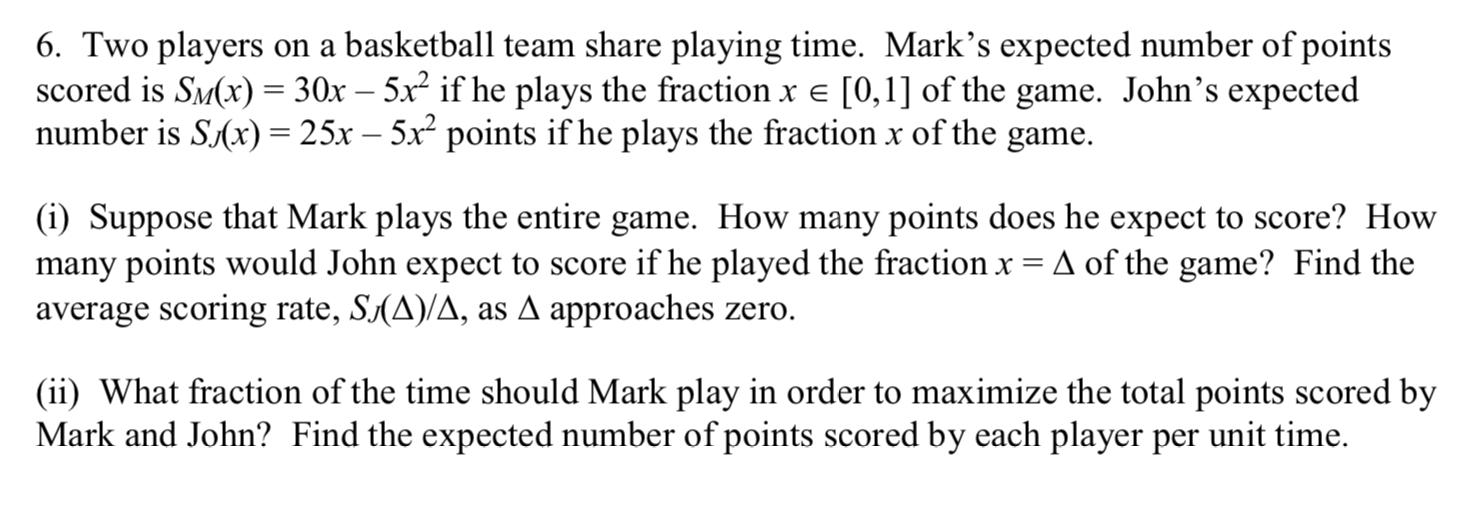help_outlineImage Transcriptionclose6. Two players on a basketball team share playing time. Mark's expected number of points scored is SM(x) = 30x – 5x² if he plays the fraction x e [0,1] of the game. John's expected number is S(x) = 25x – 5x points if he plays the fraction x of the game. (i) Suppose that Mark plays the entire game. How many points does he expect to score? How many points would John expect to score if he played the fraction x = A of the game? Find the average scoring rate, S(A)/A, as A approaches zero. (ii) What fraction of the time should Mark play in order to maximize the total points scored by Mark and John? Find the expected number of points scored by each player per unit time. fullscreen
check_circle

Step 1

(i) If Mark plays the entire game, then x = 1. Hence, the expected score is: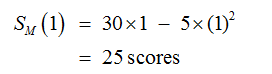If John played the fraction x = Δ, his expected score is: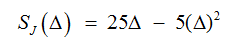Step 2

Average scoring rate can be calculated as: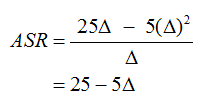As Δ approaches to 0, putting Δ = 0 in above equation,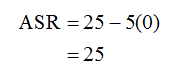Hence, ASR is 25.

Step 3

(ii) If Mark spends x fraction of time, John would spend (1 – x) fraction of time.

Total score can be calculated as: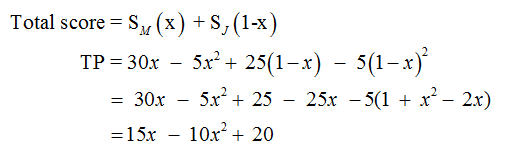For finding optimal score, differentiating using first order condition: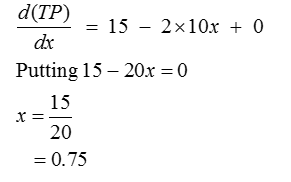Hence, Mark’s optimum play time is 75% of time.

So, John plays for 25% of time.

...

### Want to see the full answer?

See Solution

#### Want to see this answer and more?

Solutions are written by subject experts who are available 24/7. Questions are typically answered within 1 hour.*

See Solution
*Response times may vary by subject and question.
Tagged in